# Evaluate structure hypothesis

Hypotheses about an RNA structure can be tested using CLC Genomics Workbench. A structure hypothesis H is formulated using the structural constraint annotations described in Specifying structure constraints. By adding several annotations complex structural hypotheses can be formulated (see 24.21).

Given the set S of all possible structures, only a subset of these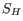will comply with the formulated hypotheses. We can now find the probability of H as: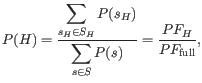where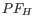is the partition function calculated for all structures permissible by H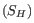and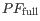is the full partition function. Calculating the probability can thus be done with two passes of the partition function calculation, one with structural constraints, and one without. 24.21.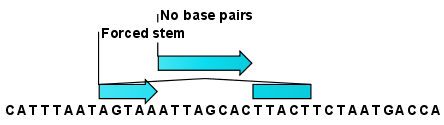Figure 24.21: Two constraints defining a structural hypothesis.

Subsections